Problem

# A square conducting loop, 20.0 cm on a side, is placed in the same magnetic field as shown...

A square conducting loop, 20.0 cm on a side, is placed in the same magnetic field as shown in Exercise 29.30. (See Fig. 29.55; the center of the square loop is at the center of the magnetic-field region.) (a) Copy Fig. 29.55, and draw vectors to show the directions and relative magnitudes of the induced electric field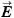at points a, b, and c. (b) Prove that the component of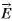along the loop has the same value at every point of the loop and is equal to that of the ring shown in Fig. 29.31 (see Exercise 29.30). (c) What current is induced in the loop if its resistance is 1.90 Ω? (d) What is the potential difference between points a and b?

Figure 29.55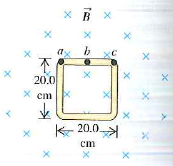Exercise 29.30

The magnetic field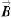at all points within the colored circle shown in Fig. 29.31 has an initial magnitude of 0.750 T. (The circle could represent approximately the space inside a long, thin solenoid.) The magnetic field is directed into the plane of the diagram and is decreasing at the rate of −0.0350 T/s. (a) What is the shape of the field lines of the induced electric field shown in Fig. 29.31, within the colored circle? (b) What are the magnitude and direction of this field at any point on the circular conducting ring with radius 0.100 m? (c) What is the current in the ring if its resistance is 4.00 Ω? (d) What is the emf between points a and b on the ring? (e) If the ring is cut at some point and the ends are separated slightly, what will be the emf between the ends?

Figure. 29.31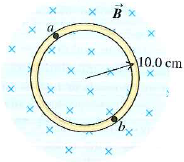#### Step-by-Step Solution

Request Professional Solution

#### Request solution!

We need 10 more requests to produce this problem solution. Share with your friends to get the problem solution faster!

0 /10 have requested this problem solution

Once 10 people have made a request, the problem solutions will be available in 1-2 days.
All students who have requested the problem solution will be notified once they are available.